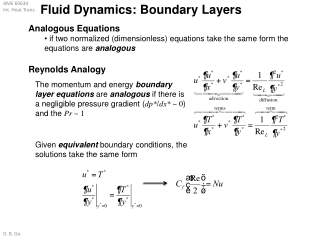DownloadDownload PresentationFluid Dynamics: Boundary Layers

# Fluid Dynamics: Boundary Layers

Télécharger la présentation## Fluid Dynamics: Boundary Layers

- - - - - - - - - - - - - - - - - - - - - - - - - - - E N D - - - - - - - - - - - - - - - - - - - - - - - - - - -
##### Presentation Transcript

1. Fluid Dynamics: Boundary Layers • Analogous Equations • if two normalized (dimensionless) equations take the same form the equations are analogous Reynolds Analogy • The momentum and energy boundary layer equations are analogous if there is a negligible pressure gradient (dp*/dx* ~ 0) and the Pr ~ 1 • Given equivalent boundary conditions, the solutions take the same form

2. Fluid Dynamics: Boundary Layers Reynolds Analogy Defining a new non-dimensional number Stanton number • The Reynolds Analogyis defined as (whenPr =1) • The Reynolds Analogyimplies that under certain conditions (no pressure gradient, Pr = 1) if the velocity parameters are known than the heat transfer parameters can be determined (and vice versa) Modified Reynolds Analogy: Chilton-Colburn (empirical) Colburn j factor laminar flows: valid fordp*/dx* ~ 0 turbulent flows: generally valid without restriction ondp*/dx*

3. External Convection: Overview average Nusselt number local Nusselt number • Determining Heat Transfer Coefficients • determining heat transfer coefficients requires an accurate knowledge of the flow field • few (pseudo-)analytical solutions exist (especially for turbulent flow) • similarity solutions, etc. • heat transfer coefficient relations are largely empiricaland are presented based on the Nusselt number • The Nusselt number (and heat transfer coefficient) are functions of the fluid properties (ν, ρ, α, c, kf) • the effect of variable properties may be considered by evaluating all properties at the film temperature • most accurate solutions often require iteration on the film properties

4. Fluid Dynamics: Boundary Layers • Laminarand turbulentboundary layers have different heat transfer characteristics • turbulent mixing typically increases heat transfer Critical Reynolds numberapproximates the location where the flow transitions from laminar to turbulent flow external (flat plate) flow internal (duct) flow • Transition

5. Fluid Dynamics: Boundary Layers Transition leads to a significant increase in the local heat transfer coefficient • Transition

6. External Convection: Overview Determining external flow conditions compute: compare to critical Reynolds number external (flat plate) flow laminar flow along length of flat plate transition to turbulent flow at critical length External Flows • boundary layers develop freely without constraint (compare to a internal/duct flow) • boundary layer may be laminar, laminar and turbulent, or entirely turbulent • simplest external flow: flat plate in parallel flow

7. External Convection: Overview laminar free stream & smooth plate Average parameters • Transition to Turbulence • critical Reynolds number affected by free stream turbulence and surface roughness of plate • nominally • if the boundary layer is “tripped” at the leading edge: • flow is turbulent along entire length of flat plate

8. External Convection: Overview delays thermal boundary layer growth • Thermal Conditions at the Surface (idealized) • uniform heat flux • uniform surface temperature • unheated starting length